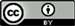1国网安徽省电力公司电力科学研究院，安徽 合肥

2国网安徽省电力公司合肥供电公司，安徽 合肥

3武汉大学电气工程学院，湖北 武汉1. 引言

2. 风电系统的调峰特性

P G - w ( t ) = P L ( t ) − P w ( t ) (1)

3. 基于粒子群算法的调峰策略优化3.1. 调峰策略的优化模型

α i = P G i max − P G i min P G i max × 100 % (2)

Minimum technical output of thermal generator

600 MW以上50
600 MW58
300 MW63
220 MW73
200 MW及以下80

min F = ∑ t = 1 T ∑ i = 1 N h C h i ( t ) + ∑ t = 1 T ∑ i = 1 N c [ [ C c i 1 ( t ) + C c i 2 ( t ) ] + ∑ t = 1 T ∑ i = 1 N w C s i ( t ) (3)

s.t.

{ P g j + Δ P G i − P l i − U i ∑ j = 1 N U j ( G i j cos θ i j + B i j sin θ i j ) = 0 Q g j − Q l i − U i ∑ j = 1 N U j ( G i j sin θ i j − B i j cos θ i j ) = 0 ∑ k = 1 N G α k P G k max ≥ ∑ m = 1 N W G Δ P m G W max + Δ P L max P g i min ≤ P g i ≤ P g i max ,       i ∈ N g U i min ≤ U i ≤ U i max ,       i ∈ N U Q g i min ≤ Q g i ≤ Q g i max , i ∈ N Q (4)

3.2. 基于粒子群算法的求解方法

{ v i ( n + 1 ) = ω × v i ( n ) + c 1 × r a n d ( 1 ) × ( P i − x i ( n ) )                                 + c 2 × r a n d ( 1 ) × ( x G − x i ( n ) ) x i ( n + 1 ) = x i ( n ) + v i ( n + 1 ) (5)

4. 仿真研究

5. 结论